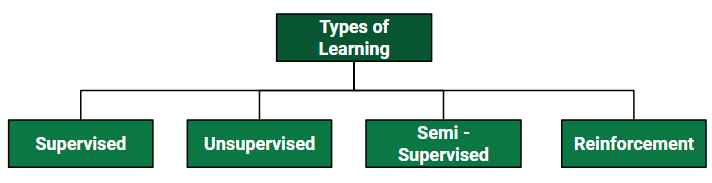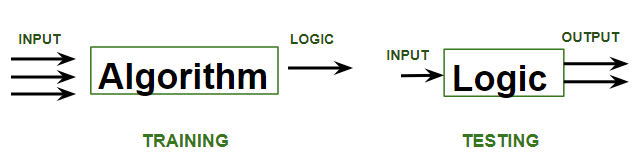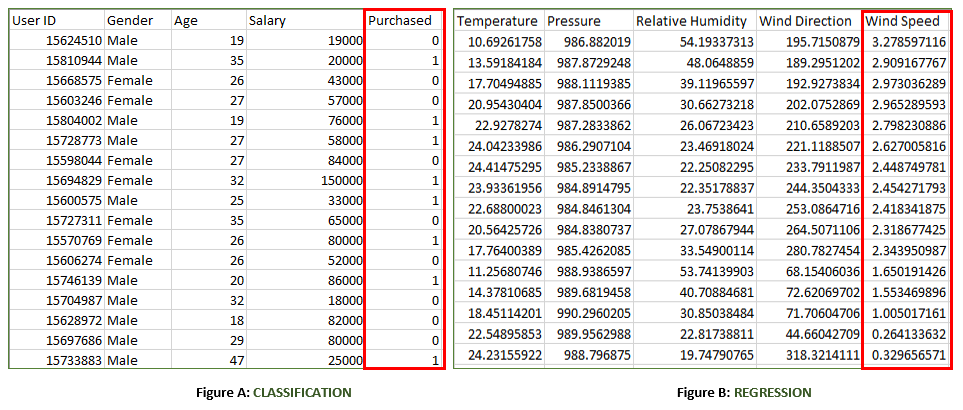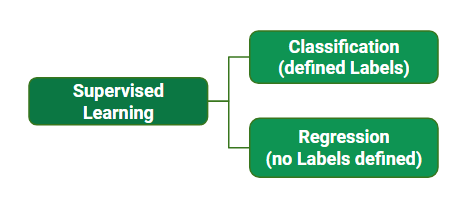Open In App

# Supervised Machine Learning

A machine is said to be learning from past Experiences(data feed-in) with respect to some class of tasks if its Performance in a given Task improves with the Experience. For example, assume that a machine has to predict whether a customer will buy a specific product let’s say “Antivirus” this year or not. The machine will do it by looking at the previous knowledge/past experiences i.e. the data of products that the customer had bought every year and if he buys an Antivirus every year, then there is a high probability that the customer is going to buy an antivirus this year as well. This is how machine learning works at the basic conceptual level.## Supervised Machine Learning

Supervised learning is a machine learning technique that is widely used in various fields such as finance, healthcare, marketing, and more. It is a form of machine learning in which the algorithm is trained on labeled data to make predictions or decisions based on the data inputs.In supervised learning, the algorithm learns a mapping between the input and output data. This mapping is learned from a labeled dataset, which consists of pairs of input and output data. The algorithm tries to learn the relationship between the input and output data so that it can make accurate predictions on new, unseen data.

Let us discuss what learning for a machine is as shown below media as follows:Supervised learning is where the model is trained on a labelled dataset. A labelled dataset is one that has both input and output parameters. In this type of learning both training and validation, datasets are labelled as shown in the figures below.

The labeled dataset used in supervised learning consists of input features and corresponding output labels. The input features are the attributes or characteristics of the data that are used to make predictions, while the output labels are the desired outcomes or targets that the algorithm tries to predict.Both the above figures have labelled data set as follows:

• Figure A: It is a dataset of a shopping store that is useful in predicting whether a customer will purchase a particular product under consideration or not based on his/ her gender, age, and salary.
Input: Gender, Age, Salary
Output: Purchased i.e. 0 or 1; 1 means yes the customer will purchase and 0 means that the customer won’t purchase it.
• Figure B: It is a Meteorological dataset that serves the purpose of predicting wind speed based on different parameters.
Input: Dew Point, Temperature, Pressure, Relative Humidity, Wind Direction
Output: Wind Speed

Training the system: While training the model, data is usually split in the ratio of 80:20 i.e. 80% as training data and the rest as testing data. In training data, we feed input as well as output for 80% of data. The model learns from training data only. We use different machine learning algorithms(which we will discuss in detail in the next articles) to build our model. Learning means that the model will build some logic of its own.
Once the model is ready then it is good to be tested. At the time of testing, the input is fed from the remaining 20% of data that the model has never seen before, the model will predict some value and we will compare it with the actual output and calculate the accuracy.

## Types of Supervised Learning Algorithm

Supervised learning is typically divided into two main categories: regression and classification. In regression, the algorithm learns to predict a continuous output value, such as the price of a house or the temperature of a city. In classification, the algorithm learns to predict a categorical output variable or class label, such as whether a customer is likely to purchase a product or not.

One of the primary advantages of supervised learning is that it allows for the creation of complex models that can make accurate predictions on new data. However, supervised learning requires large amounts of labeled training data to be effective. Additionally, the quality and representativeness of the training data can have a significant impact on the accuracy of the model.
Supervised learning can be further classified into two categories:### Regression

Regression is a supervised learning technique used to predict continuous numerical values based on input features. It aims to establish a functional relationship between independent variables and a dependent variable, such as predicting house prices based on features like size, bedrooms, and location.
The goal is to minimize the difference between predicted and actual values using algorithms like Linear Regression, Decision Trees, or Neural Networks, ensuring the model captures underlying patterns in the data.

### Classification

Classification is a type of supervised learning that categorizes input data into predefined labels. It involves training a model on labeled examples to learn patterns between input features and output classes. In classification, the target variable is a categorical value. For example, classifying emails as spam or not.
The model’s goal is to generalize this learning to make accurate predictions on new, unseen data. Algorithms like Decision Trees, Support Vector Machines, and Neural Networks are commonly used for classification tasks.

NOTE: There are common Supervised Machine Learning Algorithm that can be used for both regression and classification task.

## Supervised Machine Learning Algorithm

Supervised learning can be further divided into several different types, each with its own unique characteristics and applications. Here are some of the most common types of supervised learning algorithms:

• Linear Regression: Linear regression is a type of regression algorithm that is used to predict a continuous output value. It is one of the simplest and most widely used algorithms in supervised learning. In linear regression, the algorithm tries to find a linear relationship between the input features and the output value. The output value is predicted based on the weighted sum of the input features.
• Logistic Regression: Logistic regression is a type of classification algorithm that is used to predict a binary output variable. It is commonly used in machine learning applications where the output variable is either true or false, such as in fraud detection or spam filtering. In logistic regression, the algorithm tries to find a linear relationship between the input features and the output variable. The output variable is then transformed using a logistic function to produce a probability value between 0 and 1.
• Decision Trees: Decision tree is a tree-like structure that is used to model decisions and their possible consequences. Each internal node in the tree represents a decision, while each leaf node represents a possible outcome. Decision trees can be used to model complex relationships between input features and output variables.
A decision tree is a type of algorithm that is used for both classification and regression tasks.
• Decision Trees Regression: Decision Trees can be utilized for regression tasks by predicting the value linked with a leaf node.
• Decision Trees Classification: Random Forest is a machine learning algorithm that uses multiple decision trees to improve classification and prevent overfitting.
• Random Forests: Random forests are made up of multiple decision trees that work together to make predictions. Each tree in the forest is trained on a different subset of the input features and data. The final prediction is made by aggregating the predictions of all the trees in the forest.
Random forests are an ensemble learning technique that is used for both classification and regression tasks.
• Random Forest Regression: It combines multiple decision trees to reduce overfitting and improve prediction accuracy.
• Random Forest Classifier: Combines several decision trees to improve the accuracy of classification while minimizing overfitting.
• Support Vector Machine(SVM): The SVM algorithm creates a hyperplane to segregate n-dimensional space into classes and identify the correct category of new data points. The extreme cases that help create the hyperplane are called support vectors, hence the name Support Vector Machine.
A Support Vector Machine is a type of algorithm that is used for both classification and regression tasks
• Support Vector Regression: It is a extension of Support Vector Machines (SVM) used for predicting continuous values.
• Support Vector Classifier: It aims to find the best hyperplane that maximizes the margin between data points of different classes.
• K-Nearest Neighbors (KNN): KNN works by finding k training examples closest to a given input and then predicts the class or value based on the majority class or average value of these neighbors. The performance of KNN can be influenced by the choice of k and the distance metric used to measure proximity. However, it is intuitive but can be sensitive to noisy data and requires careful selection of k for optimal results.
A K-Nearest Neighbors (KNN) is a type of algorithm that is used for both classification and regression tasks.
• K-Nearest Neighbors Regression: It predicts continuous values by averaging the outputs of the k closest neighbors.
• K-Nearest Neighbors Classification: Data points are classified based on the majority class of their k closest neighbors.
• Gradient Boosting: Gradient Boosting combines weak learners, like decision trees, to create a strong model. It iteratively builds new models that correct errors made by previous ones. Each new model is trained to minimize residual errors, resulting in a powerful predictor capable of handling complex data relationships.
A Gradient Boosting is a type of algorithm that is used for both classification and regression tasks.
• Gradient Boosting Regression: It builds an ensemble of weak learners to improve prediction accuracy through iterative training.
• Gradient Boosting Classification: Creates a group of classifiers to continually enhance the accuracy of predictions through iterations

The power of supervised learning lies in its ability to accurately predict patterns and make data-driven decisions across a variety of applications. Here are some advantages listed below:

1. Labeled training data benefits supervised learning by enabling models to accurately learn patterns and relationships between inputs and outputs.
2. Supervised learning models can accurately predict and classify new data.
3. Supervised learning has a wide range of applications, including classification, regression, and even more complex problems like image recognition and natural language processing.
4. Well-established evaluation metrics, including accuracy, precision, recall, and F1-score, facilitate the assessment of supervised learning model performance.